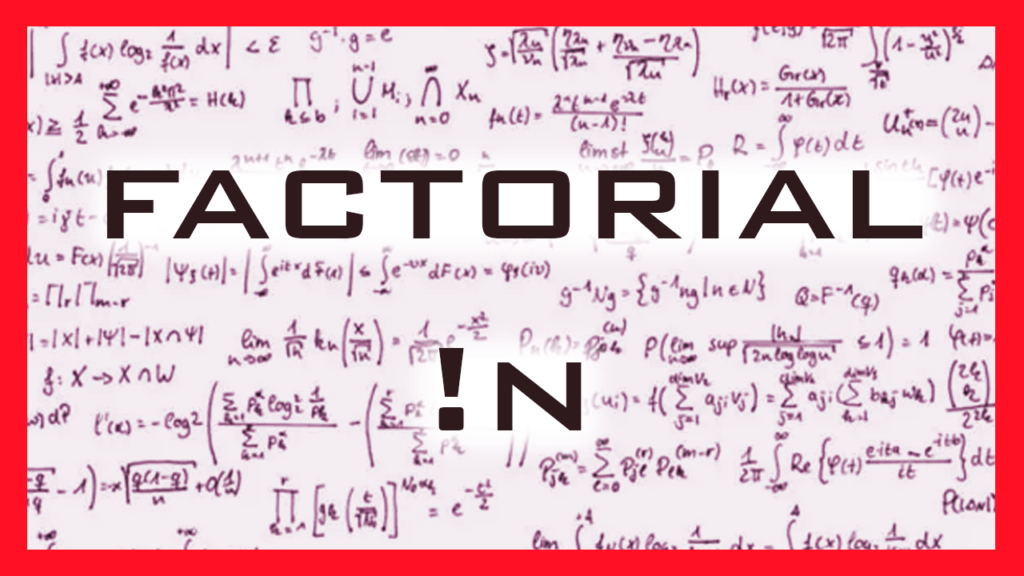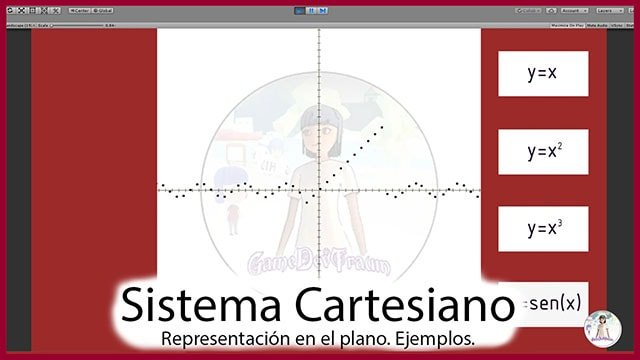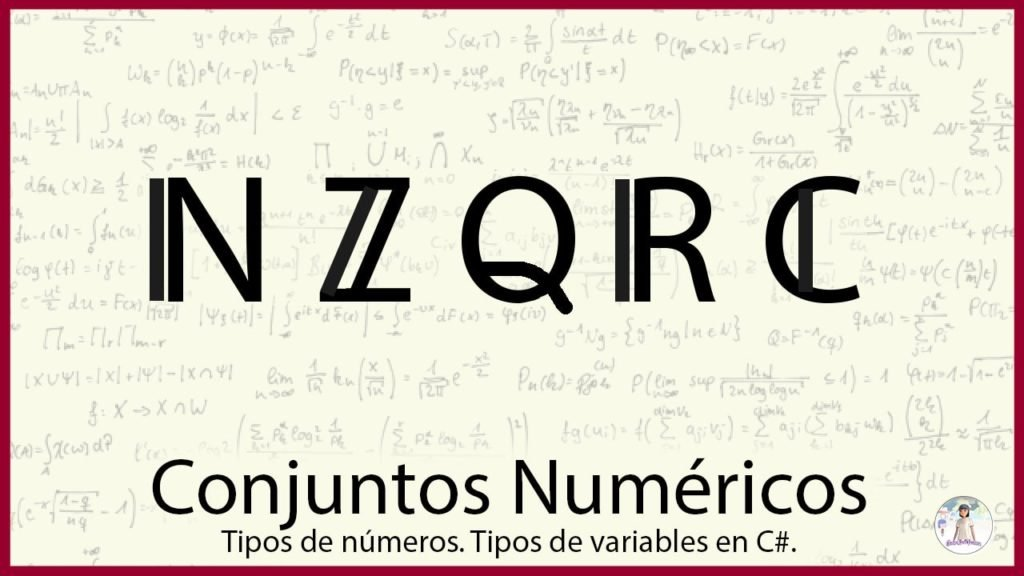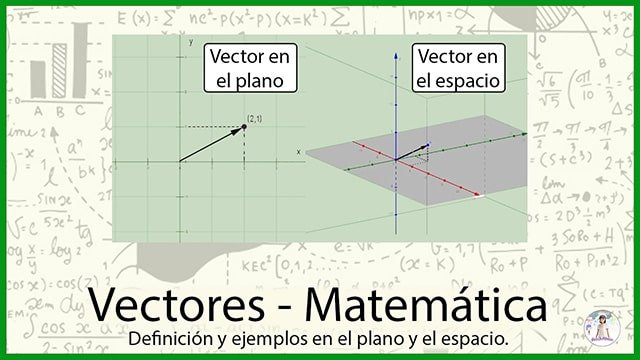# Algebra## Factorial Function

Introduction In this article we will see how to define the factorial operation of a number, calculations and properties. It is a useful operation that is commonly applied in the development in series of functions. Definition of the Factorial Function This operation is symbolized with the admiration symbol in front of the number to apply …

## Absolute Value of a Number

Introduction In this article we are going to see the absolute value function of a number, what are its properties, some graphics and as an extra we are going to see how to calculate the absolute value of an expression in the Unity graphic engine, in the field of programming and game development. Definition of …

## Summation and Product Notation

Introduction In this article we are going to talk about the summation and product notation, what they mean and how to perform the calculations. At the end we will see how to program summation and product notation in Java or C# language. What is summation and product notation? Summation and product are ways of defining …## Cartesian Plane – Coordinate System

Introduction The Cartesian coordinate system is named after the philosopher and mathematician René Descartes, considered the creator of analytical geometry. This system allows us to represent points on a line, in the plane and in space using arrays of numbers. For example: (1,5), (-3,0), (4,1,-1), etc. What is a Cartesian plane? The Cartesian plane is …## Numerical sets. Types of numbers and application

Introduction Numeric sets are used to group numbers that have similar characteristics. It is one of the basic concepts of mathematics so it is important to understand what they are and what characteristics each has. What are number sets used for? Numeric sets are used to separate numbers into different classes that have similar properties. …## Vector – Mathematics

Introduction A vector is a mathematical tool that allows us to represent magnitudes in which not only the intensity (or modulus), but also the direction and direction in which they are applied are important. The vectors are very useful in the development of games, they allow us to define directions for movement, do ray tracing, …